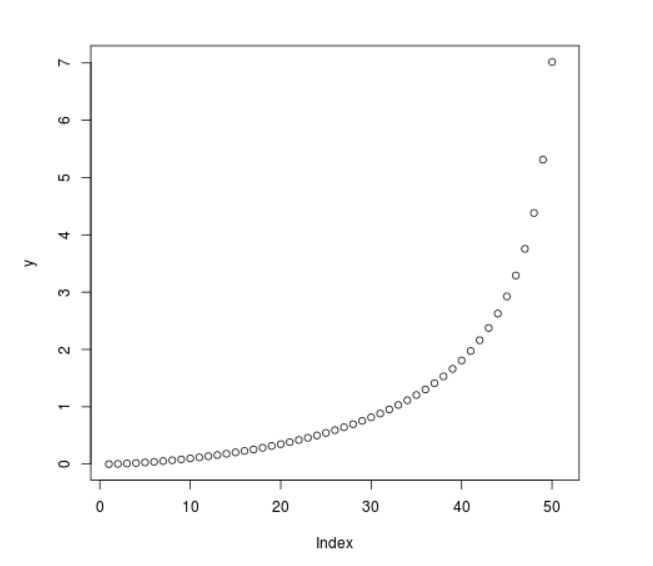Open in App
Not now

# Compute the Value of Quantile Function over Weibull Distribution in R Programming – qweibull() Function

• Last Updated : 25 Jun, 2020

`qweibull()` function in R Language is used to compute the value of Quantile Function for Weibull Distribution.

Syntax: qweibull(x, shape)

Parameters:
x: Numeric Vector
shape: Shape Parameter

Example 1:

 `# R Program to compute the value of``# Quantile Weibull Function`` ` `# Creating a sequence of x-values``x <``-` `seq(``0``, ``1``, by ``=` `0.2``)`` ` `# Calling qweibull() Function``y <``-` `qweibull(x, shape ``=` `0.5``)``y`

Output:

``` 0.00000000 0.04979304 0.26094282 0.83958871 2.59029039        Inf
```

Example 2:

 `# R Program to compute the value of``# Quantile Weibull Function`` ` `# Creating a sequence of x-values``x <``-` `seq(``0``, ``1``, by ``=` `0.02``)`` ` `# Calling qweibull() Function``y <``-` `qweibull(x, shape ``=` `0.7``)`` ` `# Plot a graph``plot(y)`

Output:My Personal Notes arrow_drop_up